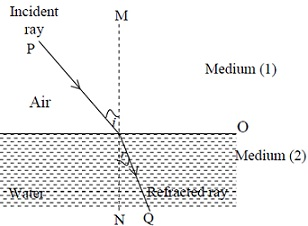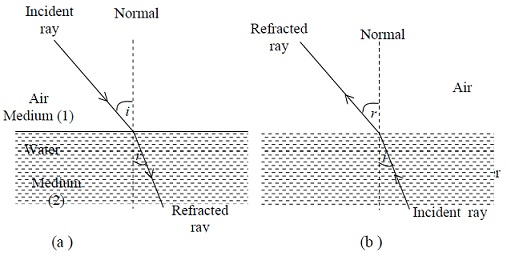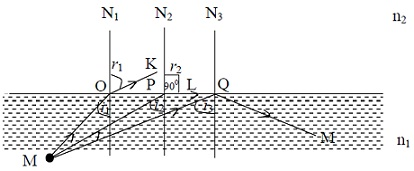#### Refraction at Plane Surfaces, Physics tutorial

Introduction:

When a ray of light passes from one medium to another through a surface (transparent), the ray bends at the surface as shown in figure given below. This bending of a ray of light is called refraction.The ray of light PO is known as incident ray while OQ is refracted ray as shown in figure. Angle r is known as angle of refraction that is formed between refracted ray OQ with normal at MN at O. Angle of refraction, depends on properties of two media in which ray travel and also on incident angle i. Medium like air is known as rarer medium and medium like glass and water are denser medium. Consider the two cases:

1. First, when the ray of light enters towards the medium where speed of light is less that is ray travels from air (medium 1) to water or glass (medium 2). Speed of light (3x108) is more in comparison to speed when it enters the block of glass (2x 108 m/s).

2. Second, when ray of light travels towards the medium where speed of light is more i.e. ray travels from water or glass (medium 2) to air (medium 1). Thus, ray is entering from the medium to second medium where the speed is greater.

Case 1:

Bending of the Ray of Light when it travels from Air to Water:

In this case, when the ray of light enters towards the medium where speed of light is less (denser medium) that is from air to glass or water, it bends towards normal as shown in (a) figure given below.

Case 2:

Bending of the Ray of Light when it travels from Water to Air:

For this situation, when the ray of light travels towards the medium where speed of light is more that is a ray of light moves from glass or water to air, then ray goes (bend) away from normal as shown (b) in figure.Laws of Refraction:

You have learnt about the angle of incidence i and angle of refraction r with the normal MN.

Snell's Law:

The relation between angle of incidence and angle of refraction was established by the scientist Snell and called as Snell's law. According to law, the sine of angle of incidence (i) and refraction (r) have a constant ratio to each other. Two laws of refraction are:

First Law:

Incident ray, refracted ray and normal at the point of incidence, all lie in same plane.

Second Law:

Ratio of sine of angle of incidence (i) to sine of angle of refraction is the constant for two given media. Mathematically, it can be stated as

sin i/sin r = constant

This equation is known as Snell's law.

Refractive Index:

The speed of light is different for different substances such as air, water, and glass. Let us consider that speed of light in vacuum (air) is c and speed of light in some substance (that is water) is v. Thus, there is relation between c and v due to difference in speed of light in the substances and can be denoted by the symbol n known as refractive index. Thus, refractive index can be stated as ratio of speed of light c in a vacuum (air) to speed of light v in some other substance. Mathematically, it can be stated as

n = c/v

In general, for two given media, if v1 is the velocity of light in medium 1 and v2 is the velocity of light in medium 2, then the refractive index n can be written as

n = speed of light in medium 1/speed of light in medium

2 = v1/v2

As, refractive index n is the ratio of speed in two different mediums; thus it is the dimensionless number and is always greater than unity as v is always less than c.

Critical Angle:

When light rays passes from water (or glass) to air (it signifies that ray is passing in medium of lower refractive index), then ray of light bends away from normal. In this given figure N1, N2 and N3 are normals at point O, P and Q respectively. MO, MP and MQ are incident rays. When the incident ray of light MO strikes the surface at O, the refracted ray is OK with angle of refraction r1. But as angle of incidence in water gets larger, so does angle of refraction.But at the particular incident angle, angle of refraction will be 90o as shown in figure. Here, for (light ray MP) angle of incidence i2, the angle of refraction r2 = 90o, refracted ray travel along surface in this case. Therefore, incident angle for which angle of refraction is 90o is known as critical angle. Notation for critical angle is Θc.

Hence, critical angle Θc is an angle of incidence for which angle of refraction is 90o. Mathematical relation between critical angle and refractive index is sin Θc = n where n is refractive index of medium.

Total Internal Reflection:

For incident angle less than Θc, there will be the refracted ray. So, it is interesting to know what occurs to rays of light, if they fall at the incidence angle greater than Θc. But for incident ray MQ at Q for which angle of incidence is i3. This angle of incidence is greater than Θc (i3 greater than Θc). It can be seen that ray is reflected back inside water. There is no refracted ray but all light is reflected back. Thus, When the ray of light incident at the angle greater than critical angle Θc, it reflects back inside medium (with the larger refractive index). This phenomenon is known as total internal reflection.

Applications of Total Internal Reflection

Total internal reflection happens only when light strikes the boundary where medium beyond is optically has the lower refractive index. Total internal reflection has wider applications.

• It is utilized in several optical instruments such as binoculars.
• Principle behind fiber optics is the total internal reflection. Through fiber optics, light can be transmitted with approximately no loss. The bundle of such light fibers is known as light pipe that can be utilized in human body, in medicines, and in communication signals.
• Images can be transferred from one point to another simply
• In surgery, the fiber optic devices are very useful to place areas of body that are not available simply. In examine internal organs of body, these are utilized.
• Another application of total internal reflection is in submarine periscope.

Tutorsglobe: A way to secure high grade in your curriculum (Online Tutoring)

Expand your confidence, grow study skills and improve your grades.

Since 2009, Tutorsglobe has proactively helped millions of students to get better grades in school, college or university and score well in competitive tests with live, one-on-one online tutoring.

Using an advanced developed tutoring system providing little or no wait time, the students are connected on-demand with a tutor at www.tutorsglobe.com. Students work one-on-one, in real-time with a tutor, communicating and studying using a virtual whiteboard technology.  Scientific and mathematical notation, symbols, geometric figures, graphing and freehand drawing can be rendered quickly and easily in the advanced whiteboard.

Free to know our price and packages for online physics tutoring. Chat with us or submit request at info@tutorsglobe.com